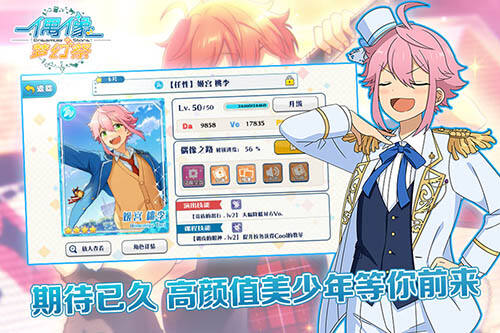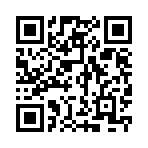# 偶像梦幻祭相性测试对应角色一览

A A A A=南云鉄虎

A A A B=葵裕太

A A A C=羽风薫

A A B A=大神晃牙

A A B B=羽风薫

A A B C=明星昂流

A A C A=仁兎成鸣

A A C B=天満光

A A C C=日日树渉

A B A A=姫宫桃李

A B A B=衣更真绪

B A A A=深海奏汰

B A A B=天翔院英智

B A A C=真白友也

B A B A=紫之创

B A B B=鸣上岚

B A B C=游木真

B A C A=仙石忍

B A C B=伏见弓弦

B A C C=瀬名泉

B B A A=鬼龙红郎

C A A A=乙狩アドニス

C A A B=高峯翠

C A A C=莲巳敬人

C A B A=葵裕太

C A B B=神崎飒马

C A B C=守沢千秋

C A C A=朔间零

C A C B=朱桜司

C A C C=氷鹰北斗

C B A A=朔间凛月#### 偶像梦幻祭

• 支持系统：
• 快捷入口：礼包 专区
• 游戏类型：模拟经营
• 游戏语言：简体中文
• 游戏下载：10227
• 游戏特征：养成,宅男,动漫